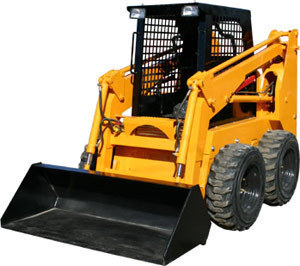•A skid loader (also called a skid steer loader) is a machinefitted with numerous arms. Various kinds of attachments may be added to the equipment. These machines can be used in various ways in construction sites.

Model 463 has an operating weight of 2,708 lbs and standard pump flow of 10 gpm. The net power is 15.7 hp. The height to bucket pin is 94.5" and the rated op. load is 700 lbs.

Model 553 has a rated op. load of 950 lbs, while the height to bucket pin is 103.7". The gross power is 26 hp and the net power is 25 hp. The standard pump flow is 11 gpm. The operating weight is 3,704 lbs.

Model 753 G has a 1,300 lb rated op. load and the net power is 43.5 hp. The standard pump flow is 14.3 gpm. The operating weight is 4,740 lbs.

Model 763 G has a rated op. load of 1,500 lbs and the height to bucket pin is 114.5". The gross power is 49 hp and net power is 46 hp. The standard pump flow is 16.7 gpm while the operating weight is 5,368 lbs.

Model S130 has a net power of 46 hp and operating weight of 4,740 lbs. The standard pump flow is 16.9 gpm. The height to bucket pin is 109.1".